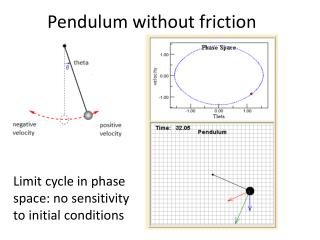DownloadDownload PresentationPendulum without friction

# Pendulum without friction

Download Presentation## Pendulum without friction

- - - - - - - - - - - - - - - - - - - - - - - - - - - E N D - - - - - - - - - - - - - - - - - - - - - - - - - - -
##### Presentation Transcript

1. Pendulum without friction Limit cycle in phase space: no sensitivity to initial conditions

2. Pendulum with friction Fixed point attractor in phase space: no sensitivity to initial conditions

3. Pendulum with friction: basin of attraction Different starting positions end up in the same fixed point. Its like rolling a marble into a basin. No matter where you start from, it ends up in the drain.

4. Pendulum with friction Adding a third dimension of potential energy: the basin of attraction as a gravitational well.

5. Inverted Pendulum: ball on flexible rod flops to one side or the other Basin of attraction in phase space: two fixed points.

6. Inverted Pendulum: ball on flexible rod Potential energy plot shows the two fixed points as the “landscape” of the basin of attraction.

7. Driven Pendulum with friction Horizontal version: http://www.youtube.com/watch?v=0LSPxDB8OPM Chaotic behavior in time

8. Driven Pendulum with friction Horizontal version: http://www.youtube.com/watch?v=0LSPxDB8OPM Chaotic attractor in phase space

9. Very simple device, but its motion can be very complex (here an LED is attached in a time exposure photo) Double Pendulum Simulation at http://www.youtube.com/watch?v=QXf95_EKS6E

10. Logistic Equation: a period-doubling route to chaos 0<x<1 (think of x as percentage of total population, say 1 million rabbits) Population this year: xt Population next year: xt+1 Rate of population increase: R Positive Feedback Loop: xt+1= R*xt Negative Feedback Loop: 1-xt (if x gets big, 1-x gets small)

11. Logistic Equation: a period-doubling route to chaos Positive Feedback Loop: xt+1= R*xt

12. Logistic Map Starting at xt= 0.2 and R= 2: “fixed point” or “point attractor.” All starting values are in this “basin of attraction” so they eventually end there.

13. Logistic Map Starting at xt= 0.2 and R= 3.1: limit cycle of “period two” (because it oscillates between two values).

14. Logistic Map: cobweb diagram Starting at xt= 0.2 and R= 3.1: limit cycle of “period two” (because it oscillates between two values). In each iteration there are two steps. The first gives the parabola, . The second step we “reset” xt to xt+1 which is the straight line. We see a “fixed point Attractor. Animation: http://lagrange.physics.drexel.edu/flash/logistic/

15. Logistic Map Starting at xt= 0.2 and R = 3.49 we double the period (“bifurcation”): a limit cycle of four values.

16. Logistic Map Increasing R continues to double the period. Starting at xt= 0.2 and R = 4 we see a chaotic attractor. The values will never repeat.

17. Bifurcation Map Where does x “settle to” for increasing R values?

18. Bifurcation Map The logistic map is a fractal: similar structure at different scales. Thus bifurcations happen with increasing frequency: the rate of increase is the Feigenbaum constant (4.7)

19. Water drop model Plotting the time interval between one drip and the next: The amount of water in a drip depends on the drip that came before it—this feedback can create complex dynamics. Tn+1 Tn Two frequency drip One-frequency drip The period-doubling route to chaos: eventually the dripping faucet produces a strange attractor: